# RBSE Maths Class 10 Chapter 8: Heights And Distance Important Questions and Solutions

RBSE Class 10 Maths Chapter 8 – Heights and Distance Important questions and solutions are available here. All these questions contain detailed explanations, along with their images, for a better understanding of the solutions. The RBSE Class 10 important questions and solutions provided at BYJU’S will help the students in scoring maximum marks.

Chapter 8 of RBSE Class 10 has only one exercise which contains questions involving the applications of trigonometry, such as finding the height of tower, hill, pole, building and so on. All these questions involve two major concepts called the angle of elevation and angle of depression. These concepts will help in finding the required measure in that particular situation when combined with trigonometric ratios.

### RBSE Maths Chapter 8: Exercise 8.1 Textbook Important Questions and Solutions

Question 1: The length of the shadow of a vertical pillar is equal to the height of pillar, then angle of elevation of Sun will be:

(a) 45°

(b) 30°

(c) 60°

(d) 50°

Solution:

Let BC be the tower and AB be its shadow.

And θ be the angle of elevation of Sun,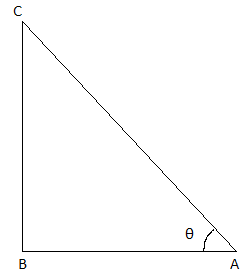According to the given,

AB = BC

In right triangle ABC,

tan θ = BC/AB = BC/BC = 1 = tan 45°

Thus, θ = 45°

Therefore, the angle of elevation is 45°.

Question 2: From a point on the ground which is 100 m away from the foot of the tower, the angle of elevation of the top of the tower is 60°, then height of tower is:

(a) 100√3 m

(b) 100/√3 m

(c) 50√3 m

(d) 200/√3 m

Solution:

Let BC be the tower.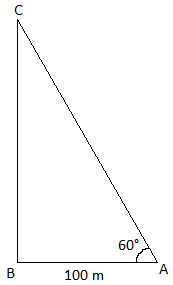Distance of a point from the foot of the tower = AB = 100 m

Angle of elevation = ∠CAB = 60°

In right triangle ABC,

tan 60° = BC/AB

√3 = BC/100

BC = 100√3

Therefore, the height of the tower is 100√3 m.

Question 3: A 15 m long ladder touches the top of a vertical wall. If this ladder makes an angle of 60° with the wall, then height of the wall is:

(a) 15√3 m

(b) 15√3/2 m

(c) 15/2 m

(d) 15 m

Solution:

Let BC be the wall and AC be the ladder.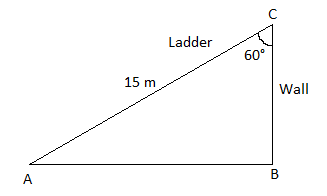AC = 15 m, angle of depression = ∠ACB = 60°

In right triangle ABC,

cos 60° = BC/AC

1/2 = BC/15

⇒ BC = 15/2

Therefore, the height of the wall is 15/2 m.

Question 4: From the top of a 10 m high tower, the angle of depression at a point on earth is 30°. Distance of point from base of tower is

(a) 10√3 m

(b) 10/√3 m

(c) 10 m

(d) 5√3 m

Solution:

Let BC be the tower.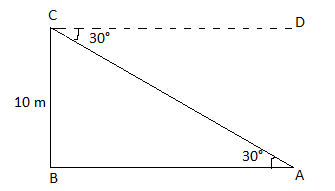Distance of a point (A) from the base of tower = AB

Angle of depression = 30°

Thus, ∠DCA = ∠CAB = 30°

In right triangle ABC,

tan 30° = BC/AB

1/√3 = 10/AB

⇒ AB = 10√3

Therefore, the required distance is 10√3 m.

Question 5: A bridge above the river makes an angle of 45° with the bank of the river. If length of bridge above the river is 150 m, then breadth of river will be

(a) 75 m

(b) 50√2 m

(c) 150 m

(d) 75√2 m

Solution:

Let AC be the length of the bridge.

BC be the bank of the river and AB be the breadth of the river.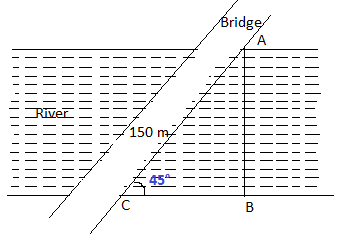Angle of elevation = ∠ACB = 45°

sin 45° = AB/AC

1/√2 = AB/150

AB = 150/√2

AB = (150/√2) × (√2/√2)

AB = (150√2)/2

AB = 75√2 m

Therefore, the breadth of the river is 75√2 m.

Question 6: Tops of two towers of height 20 m and 14 m are joined by a wire. If the wire makes an angle of 30° with horizontal line, then length of wire is:

(a) 12 m

(b) 10 m

(c) 8 m

(d) 6 m

Solution:

Let AB and CD be the two towers.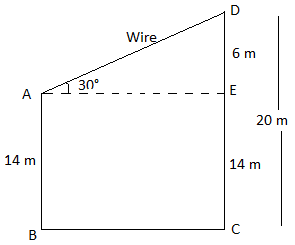In right triangle AED,

⇒ AD = 6 × 2 = 12

Therefore, the length of the wire is 12 m.

Question 7: The angle of elevation if the top of the tower from two points distance a and b from the base of tower (a > b) are 30° and 60°, then height of tower is:

(a) √(a + b)

(b) √(a – b)

(c) √(ab)

(d) √(a/b)

Solution:

Let AB be the tower.

C and D be the points of observation.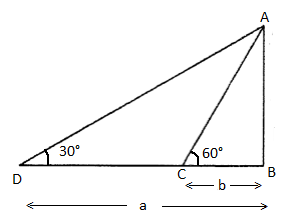∠ACB = 60°

In right triangle ABC,

tan 60° = AB/BC

√3 = AB/b

⇒ AB = b√3 ….(i)

In right triangle ABD,

tan 30° = AB/BD

1/√3 = AB/a

⇒ AB = a/√3 ….(ii)

Multiplying (i) and (ii),

AB × AB = (b√3) × (a/√3)

AB2 = ab

AB = √(ab)

Therefore, the height of the tower is √(ab).

Question 8: From the top of a 25 m high pillar, the angle of elevation of the top of the tower is same as the angle of depression of foot of tower, then height of tower is:

(a) 25 m

(b) 100 m

(c) 75 m

(d) 50 m

Solution:

Let AB be the pillar and CE be the tower.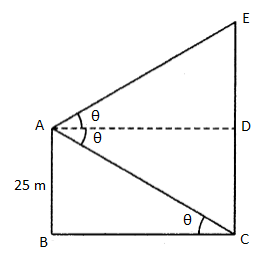∠DAE = ∠DAC = ∠ACB = θ

AB = CD = 25 m

In right triangle ABC,

tan θ = AB/BC

tan θ = 25/BC

BC = 25/tan θ

BC = 25 cot θ ….(i)

tan θ = DE/25 cot θ [From (i)]

DE = 25 × cot θ × tan θ

DE = 25

CE = CD + DE = 25 + 25 = 50

Therefore, the height of the tower is 50 m.

Question 9: If the ratio of length of a vertical rod and length of its shadow is 1 : √3, then angle of elevation of Sun is:

(a) 30°

(b) 45°

(c) 60°

(d) 90°

Solution:

Let AB be the length of the rod and BC be its shadow.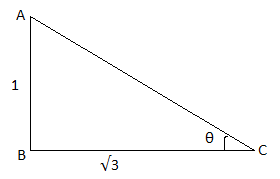Angle of elevation = θ

In right triangle ABC,

tan θ = AB/BC

tan θ = 1/√3

tan θ = tan 30°

⇒ θ = 30°

Therefore, the angle of elevation is 30.

Question 10: The slope of a hill makes an angle of 60° with horizontal. If to reach at top, 500 m distance has to be covered, then height of the hill is:

(a) 500√3 m

(b) 500/√3 m

(c) 250√3 m

(d) 250/√3 m

Solution:

Let AB be the hill and AC be its slope.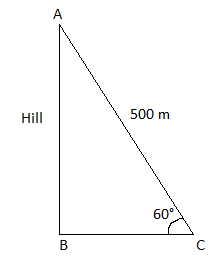Angle of elevation = ∠ACB = 60°

In right triangle ABC,

sin 60° = AB/AC

√3/2 = AB/500

⇒ AB = 500 × (√3/2)

⇒ AB = 250√3

Therefore, the height of the hill is 250√3 m.

Question 11: From a point on the ground which is 120 m away from the foot of the unfinished tower, the angle of elevation of the top of the tower is found to be 30°. Find how much the height of the tower has to increase so that its angle of elevation at the same point becomes 60°.

Solution: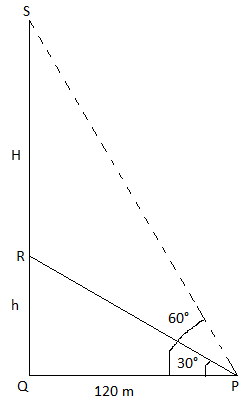Let QR be an unfinished tower of height h.

Point P is at 120 m distance from the base of the tower Q and the angle of elevation is 30° from its top (R), i.e. ∠RPQ = 30°.

Let QS be the tower height H till point S so that the angle of elevation becomes 60° from point P.

In right triangle PQR,

tan 30° = QR/PQ

1/√3 = h/120

⇒ h = 120/√3

⇒ h = (120/√3) × (√3/√3)

⇒ h = 40√3 m.

In right triangle PQS,

tan 60° = QS/PQ

√3 = (h + H)/120

⇒ h + H = 120√3

⇒ 40√3 + H = 120√3

⇒ H = 120√3 – 40√3

⇒ H = 80√3

Therefore, the height of the tower to be increased is 80√3 m.

Question 12: The angle of elevation of the top of a tower from a point situated at 100 m far from the foot of the tower is 30°. Find the height of the tower.

Solution:

Let AB be the height of the tower.

C be the point of observation.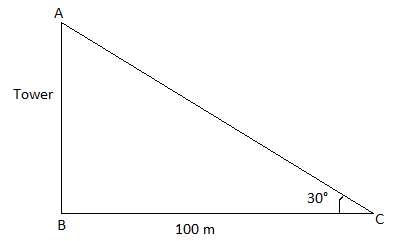Angle of elevation = ∠ACB = 30°

In right triangle ABC,

tan 30° = AB/BC

1/√3 = AB/100

⇒ AB = 100/√3

Therefore, the height of the tower is 100/√3 m.

Question 13: The angle of elevation of the top of a pillar from a point on the ground is 15° on walking 100 m towards the tower, the angle of elevation is found to be 30°. Find the height of the tower (where tan 15° = 2 – √3).

Solution:

Let AB be the pillar, C and D be the points of observation.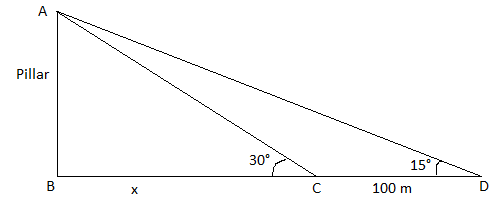The angle of elevation at point D is 15° and at C is 30°.

CD = 100 m

BC = x m

In right triangle ABC,

tan 30° = AB/BC

1/√3 = AB/x

⇒ x = AB√3 m ….(i)

In right triangle ABD,

tan 15° = AB/BD

2 – √3 = AB/(100 + x)

(2 – √3)(100 + AB√3) = AB [From (i)]

⇒ (100 + AB√3) = AB/(2 – √3)

⇒ (100 + AB√3) = AB(2 + √3)/ [(2 – √3)(2 + √3)]

⇒ 100 + AB√3 = AB(2 + √3)/(4 – 3)

⇒ 100 + AB√3 = AB(2 + √3)

⇒ AB(2 + √3) – AB√3 = 100

⇒ 2AB = 100

⇒ AB = 100/2 = 50

Therefore, the height of the pillar is 50 m.

Question 14: The shadow of a vertical tower on level ground is increased by 40 m, when the altitude of the Sun changes from 60° to 30°. Find the height of the tower.

Solution:

Let AB be the height of the tower.

C and D be the points of observation with angles of elevation 60° and 30°, respectively.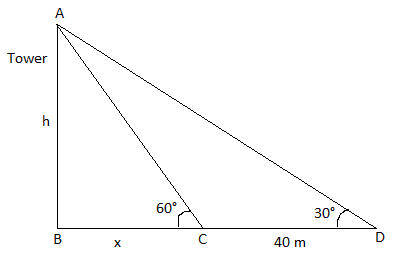AB = h m

BC = x m

CD = 40 m

In right triangle ABC,

tan 60° = AB/BC

√3 = h/x

⇒ x = h/√3 m ….(i)

In right triangle ABD,

tan 30° = AB/BD

1/√3 = AB/BD

1/√3 = h/(40 + x)

⇒ √3h = 40 + x

⇒ √3h = 40 + h/√3 [From (i)]

⇒ √3h – h/√3 = 40

⇒ h(√3 – 1/√3) = 40

⇒ h[(3 – 1)/√3] = 40

⇒ (2/√3)h = 40

⇒ h = (40√3)/2

⇒ h = 20√3 m

Therefore, the height of the tower is 20√3 m.

Question 15: From the top of a hill, in the East side at two points of angle of depression are 30° and 45°. If the distance between two points is 1 km, then find the height of the hill.

Solution:

Let AB be the hill and C, D be the two points.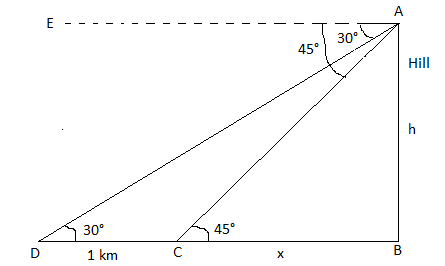Angles of depression are:

∠EAC = ∠ACB = 45°

AB = h km

BC = x km

In right triangle ABC,

tan 45° = AB/BC

1 = h/x

⇒ x = h ….(i)

In right triangle ABD,

tan 30° = AB/BD

1/√3 = AB/BD

1/√3 = h/(1 + x)

⇒ √3h = 1 + x

⇒ √3h = 1 + h [From (i)]

⇒ √3h – h = 1

⇒ h(√3 – 1) = 1

⇒ h = 1/(√3 – 1)

⇒ h = [1/(√3 – 1)] × [(√3 + 1)/(√3 + 1)]

⇒ h = (√3 + 1)/(3 – 1)

⇒ h = (√3 + 1)/2 km

Therefore, the height of the hill is (√3 + 1)/2 km.

Question 16: The angle of elevation of a cloud from a point 20 m above a lake (point A) is 30°. If the angle of depression of its reflection from point A is 60°, then find the distance of the cloud from point A.

Solution:

Let A be the point above the lake (point of observation).

C be the position of the cloud and D be its reflection in the lake.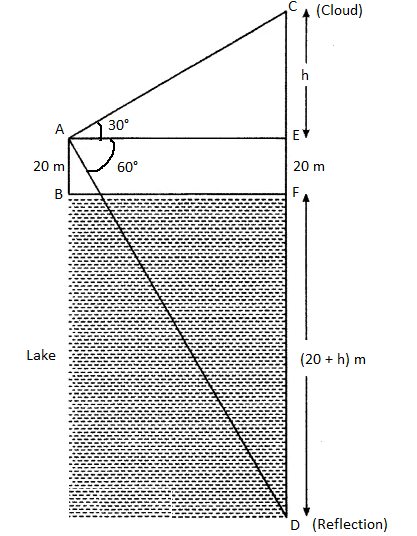∠CAE = 30°

∠DAE = 60°

AB = EF = 20 m

CE = h m

DF = (20 + h) m

DE = 20 + 20 + h = (40 + h) m

In right triangle AEC,

tan 30° = EC/AE

1/√3 = h/AE

⇒ AE = h√3 m ….(i)

In right triangle AED,

tan 60° = DE/AE

√3 = (40 + h)/AE

⇒ AE = (40 + h)/√3 ….(ii)

From (i) and (ii),

h√3 = (40 + h)/√3

h(√3 × √3) = 40 + h

3h – h = 40

2h = 40

h = 40/2 = 20 m

In right triangle AEC,

sin 30° = EC/AC

1/2 = h/AC

⇒ AC = 20 × 2 = 40 m

Therefore, the distance of the cloud from point A is 40 m.

Question 17: From a point on a bridge across a river, the angles of depression of the banks on opposite sides of the river are 30° and 45°, respectively. If the bridge is at a height of 4 m from the bank, find the width of the river.

Solution: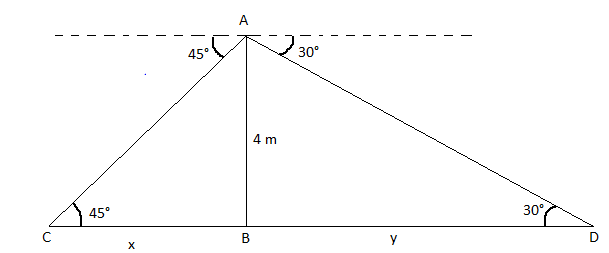Height of the bridge from the river = AB = 4 m

BC = x, BD = y

∠ACB = 45°

In right triangle ABC,

tan 45° = AB/BC

1 = 4/x

⇒ x = 4 m….(i)

In right triangle ABD,

tan 30° = AB/BD

1/√3 = 4/y

⇒ y = 4√3 m ….(ii)

From (i) and (ii),

Width of the river = x + y

= 4√3 + 4

= 4(√3 + 1) m

Therefore, the width of the river is 4(√3 + 1) m.

Question 18: A man on the deck of the ship is 10 m above water level. He observes that the angle of elevation of the top of the hill is 60° and the angle of depression of the base is 30°, then find the distance of the hill from the ship and height of the hill.

Solution:

Let AB be the hill and CD be the deck.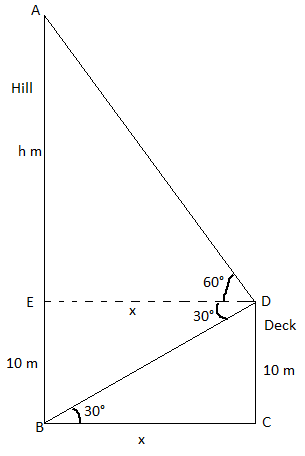In right triangle BCD,

tan 30° = CD/BC

1/√3 = 10/x

⇒ x = 10√3 m ….(i)

In right triangle AED,

tan 60° = AE/DE

√3 = h/x

√3 = h/(10√3) [From (i)]

⇒ h = (10√3) × √3

⇒ h = 30 m

Height of the hill = AB = AE + EB

= 30 + 10

= 40 m

Distance of the hill from the ship = BC = x = 10√3 m

Question 19: A vertical straight tree 12 m high is broken by strong wind in such a way that its top touches the ground and makes an angle of 60° with the ground. Find at what height from the ground did the tree break? (√3 = 1.732)

Solution:

Let AB be the tree and CD be the broken part of the tree.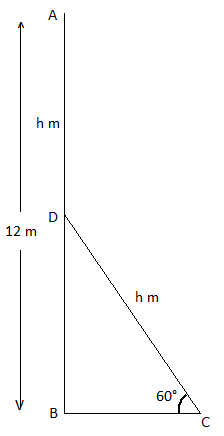AB = 12 m

AD = CD = h m

Angle of elevation = ∠DCB = 60°

Height of the remaining part of the tree = BD = (12 – h) m

In right triangle CBD,

sin 60° = BD/CD

√3/2 = (12 – h)/h

√3h = 2(12 – h)

√3h = 24 – 2h

√3h + 2h = 24

h(√3 + 2) = 24

h(1.732 + 2) = 24

h(3.732) = 24

h = 24/3.732 = 6.4308

BD = 12 – h

= 12 – 6.4308

= 5.5692

Therefore, the tree has been broken at a height of 5.5692 m from the ground.

Question 20: A tower and a building on the opposite side of the road are situated. The angles of depression from the top of the tower at the roof and base of the building are 45° and 60°, respectively. If the height of the building is 12 m, then find the height of the tower. (√3 = 1.732)

Solution:

Let AB be the tower and CD be the building.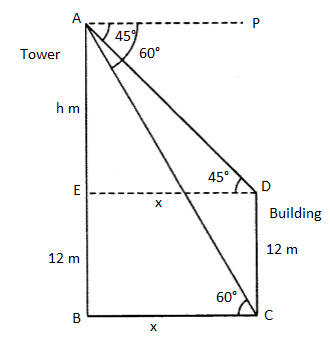In right triangle AED,

tan 45° = AE/ED

1 = h/x

h = x ….(i)

In right triangle ABC,

tan 60° = AB/BC

√3 = (12 + h)/x

√3 = (12 + h)/h [From (i)]

√3h = 12 + h

√3h – h = 12

h(√3 – 1) = 12

h(1.732 – 1) = 12

h = 12/0.732

h = 16.393 m

Height of the tower = AB = 12 + h

= 12 + 16.393

= 28.393 m

Question 21: If the angle of elevation of the Sun changes from 30° to 60°, then at these angles of elevation find the difference in the length of shadow of the 15 m high pillar.

Solution:

Let AB be the pillar.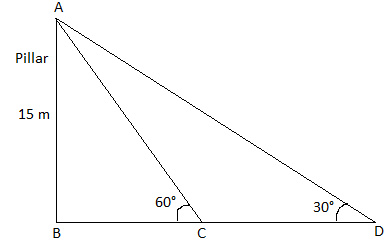In right triangle ABC,

tan 60° = AB/BC

√3 = 15/BC

⇒ BC = 15/√3

⇒ BC = (15/√3) × (√3/√3)

⇒ BC = (15√3)/3

⇒ BC = 5√3-* m

In right triangle ABD,

tan 30° = AB/BD

1/√3 = 15/BD

⇒ BD = 15√3

Difference between in length of the shadow = CD

= BD – BC

= 15√3 – 5√3

= 10√3 m

### RBSE Maths Chapter 8: Additional Important Questions and Solutions

Question 1: A kite is flying at a height of 75 metres from the level of ground attached to a string inclined at 60° to the horizontal. Find the length of the string.

Solution:

Let A be the position of the kite.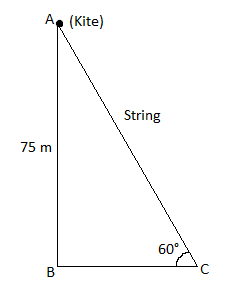AC be the string.

In right triangle ABC,

sin 60° = AB/AC

√3/2 = 75/AC

⇒ AC = 75 (2/√3)

⇒ AC = 150/√3

⇒ AC = (150/√3) (√3/√3)

⇒ AC = (150√3)/3

⇒ AC = 50√3 m

Therefore, the length of the string is 50√3 m.

Question 2: If the elevation angle of a camera situated at the top of a pole from a point 20 m away from the base of the pole is 60°, find the height of the pole.

Solution:

Let A be the position of camera and C be the point of observation.

Pole = AB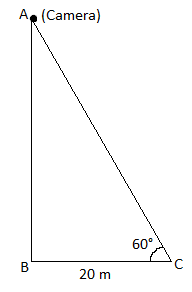In right triangle ABC,

tan 60° = AB/BC

√3 = AB/20

⇒ AB = 20/√3

Therefore, the height of the pole is 20√3 m.

Question 3: The angles of elevation of the top of a tower from two points at a distance of 9 m and 25 m from the base of the tower in the same straight line are complementary. Find the height of the tower.

Solution:

Let AB be the tower and C, D be the points of observation.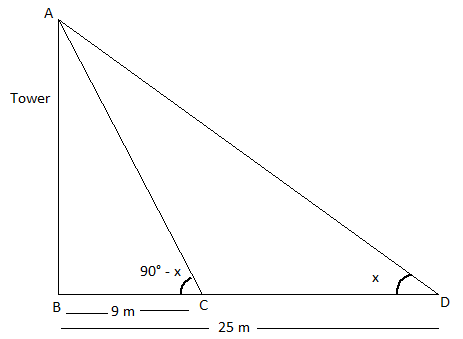In right triangle ABC,

tan (90°-x) = AB/BC

cot x = AB/9

AB = 9 cot x ….(i)

In right triangle ABD,

tan x = AB/BD

tan x = AB/25

AB = 25 tan x ….(i)

Multiplying (i) and (ii)

AB2 = 9 cot x × 25 tan x

⇒ AB2 = 225

⇒ AB = ± 15

Height cannot be negative.

Therefore, the height of the tower is 15 m.